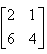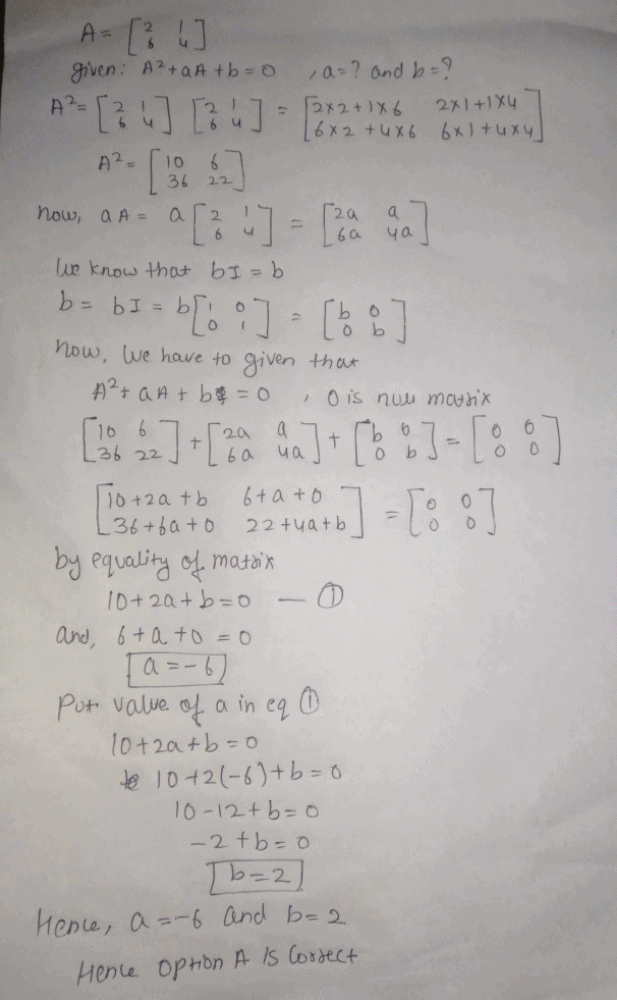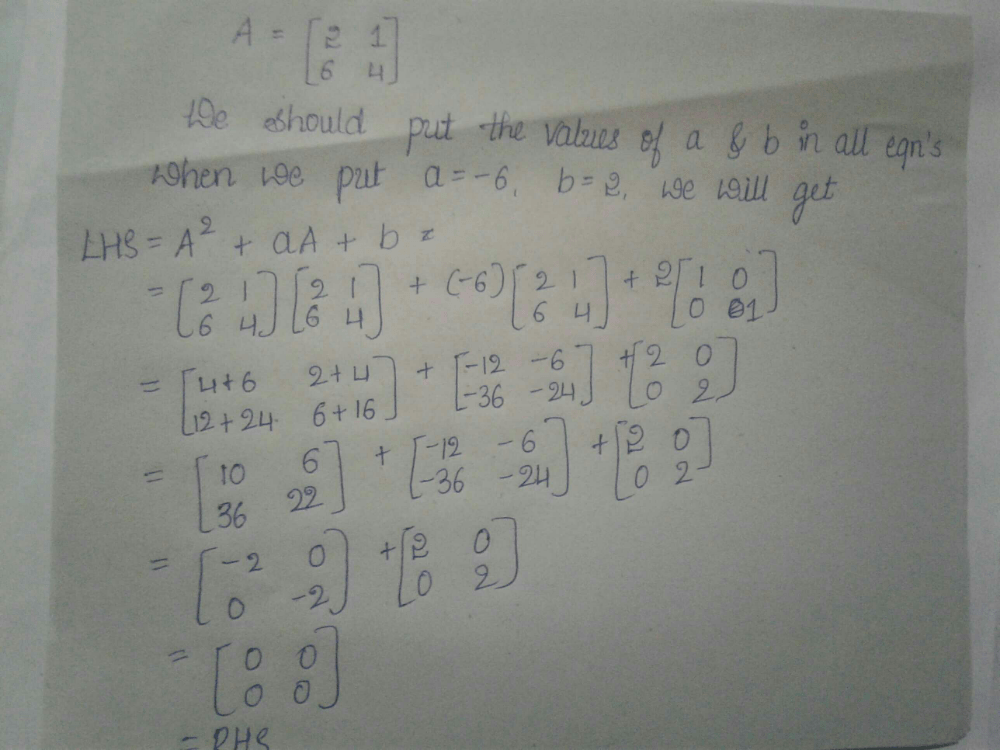Courses

# If matrix A =and A2 + aA + b = O, then the values of a and b are:a)a = – 6, b = 2b)a = 6, b = – 2c)a = 6, b = 2d)a = – 6, b = – 2Correct answer is 'A'. Can you explain this answer? Related Test: Test: Minors And Cofactors

## JEE Question

By Aman Ansari · Feb 03, 2019 ·JEE

Ananya Jha answered Jul 19, 2020Aman Choudhary answered Jul 21, 2020
ATechnical Om answered Oct 09, 2018
Ans is a=-6 and b=2 if u want full soln just text me